## 第四次作业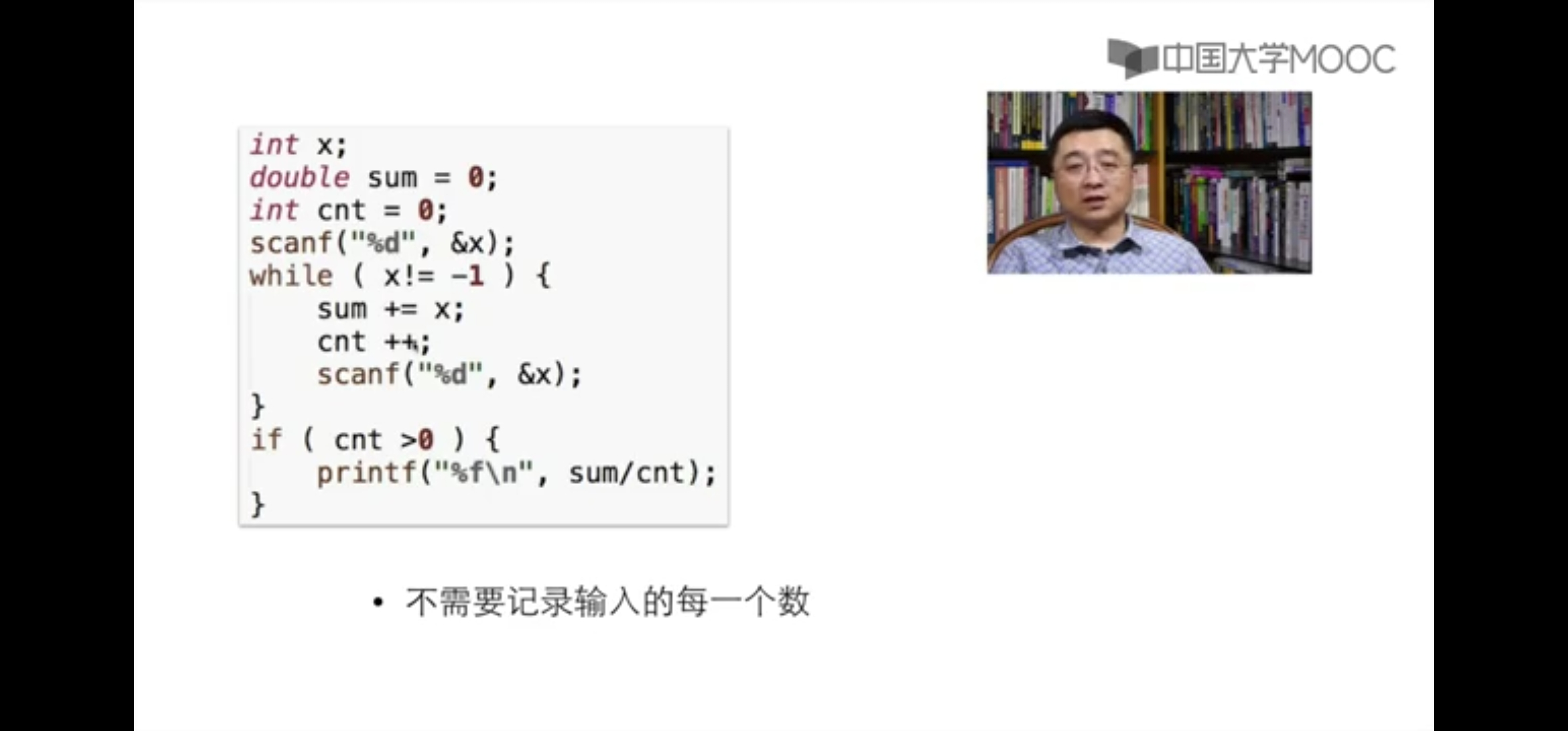1）第十三次作业：7-2 输出所有大于平均值的数 （15 分）

#include<stdio.h>
int main(){
int n,i;
double average,sum;
scanf("%d",&n);
int a[n];
if(n<1||n>10){
printf("Invalid.");
}
else{
sum = 0;
for(i=0;i<n;i++){
scanf("%d",&a[i]);
sum = sum + a[i];
}
average = sum / n;
printf("%.2f",average);
printf("\n");
for(i=0;i<n;i++){
if(a[i]>average){
printf("%d ",a[i]);
}
}
}
printf("\n");
return 0;
}

2）解题思路

1.先定义一个数组，利用for循环进行数组内的数值的赋值

2.之后进行对所输入的数值进行加和然后求出平均值

3.计算出平均值后，再次利用for循环来进行比较

4.输出平均值，以及比平均值大的数

3）流程图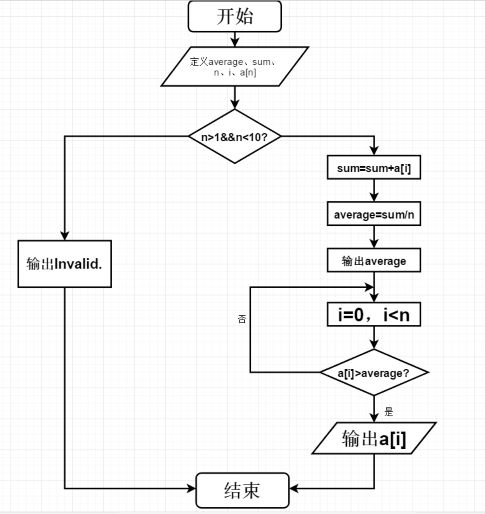4）调试过程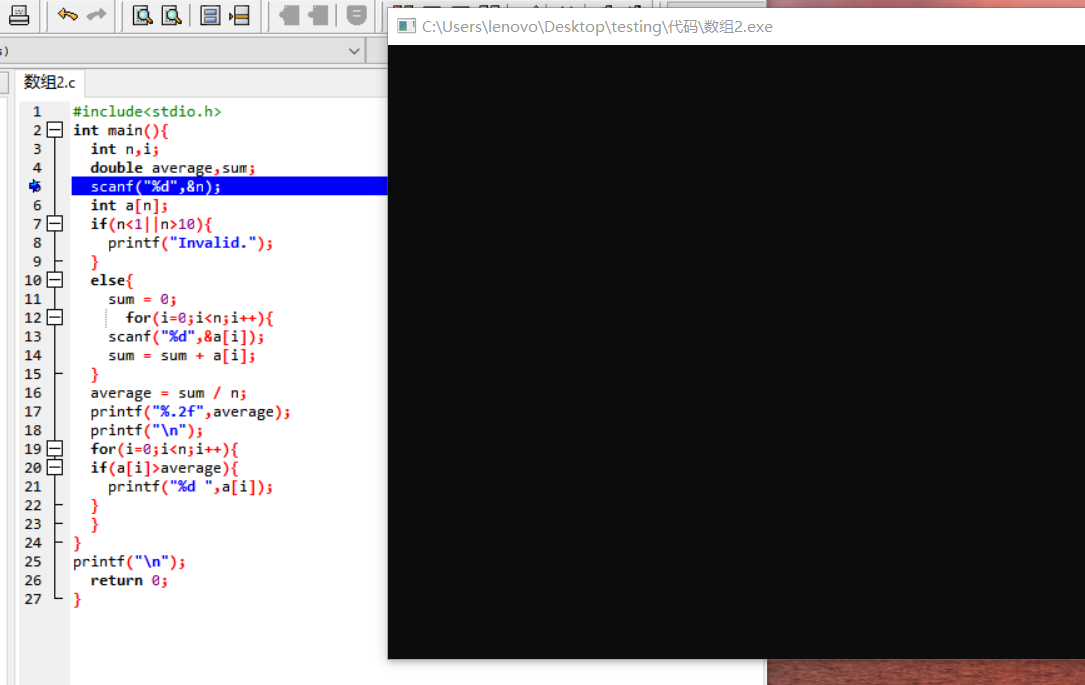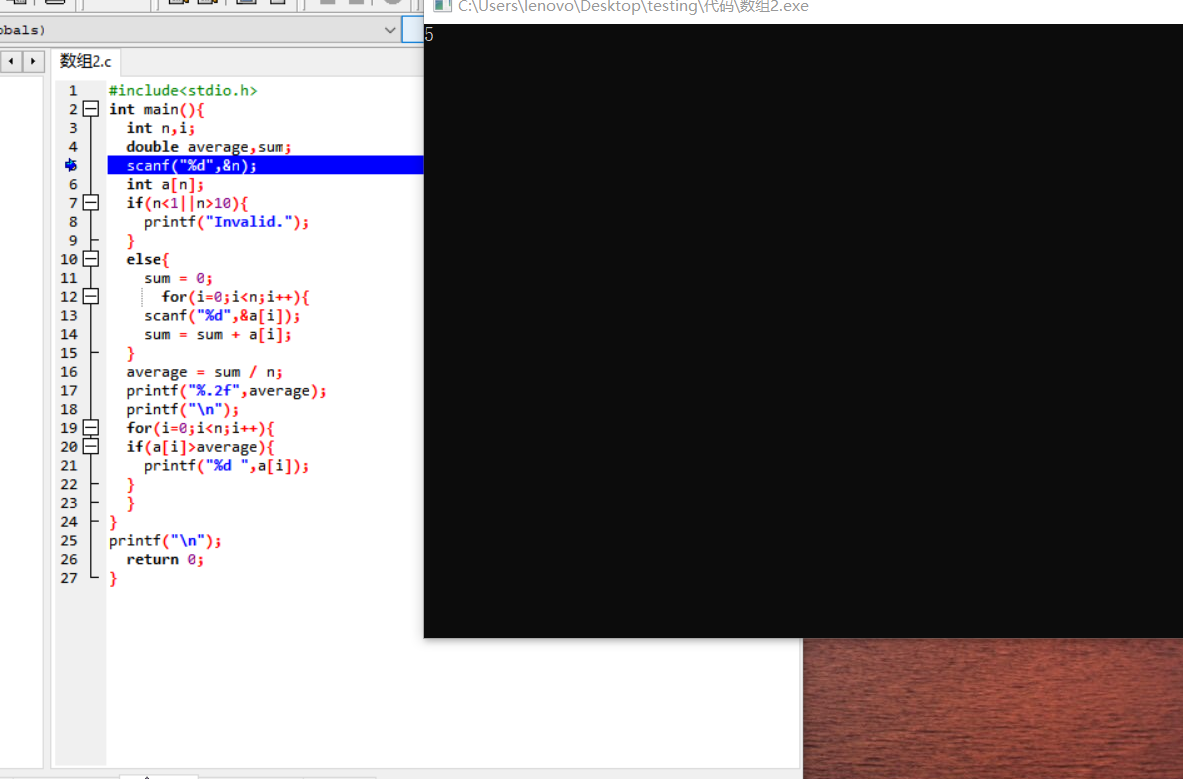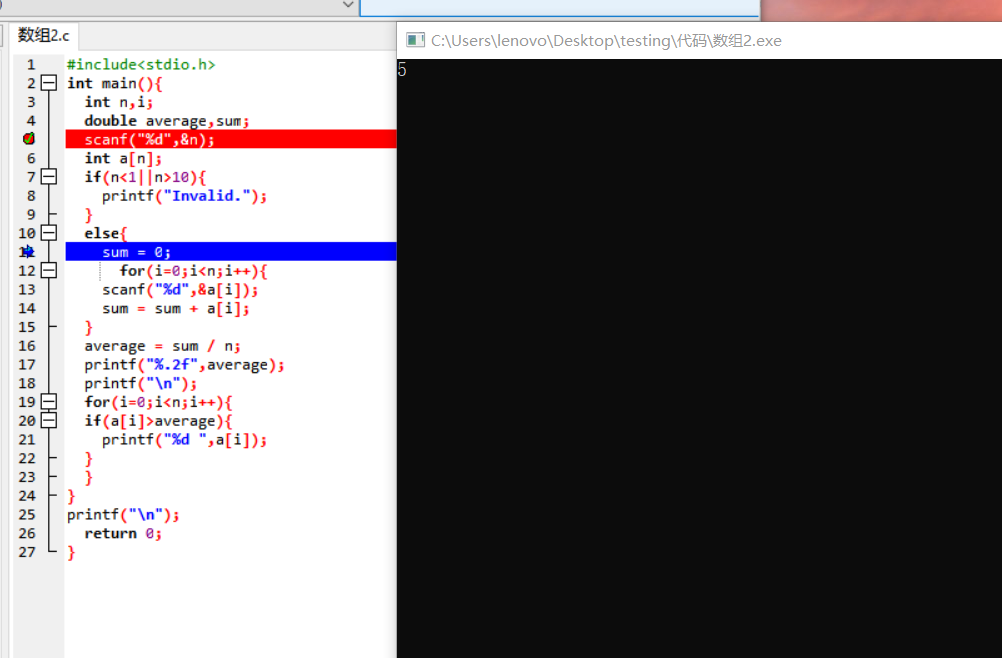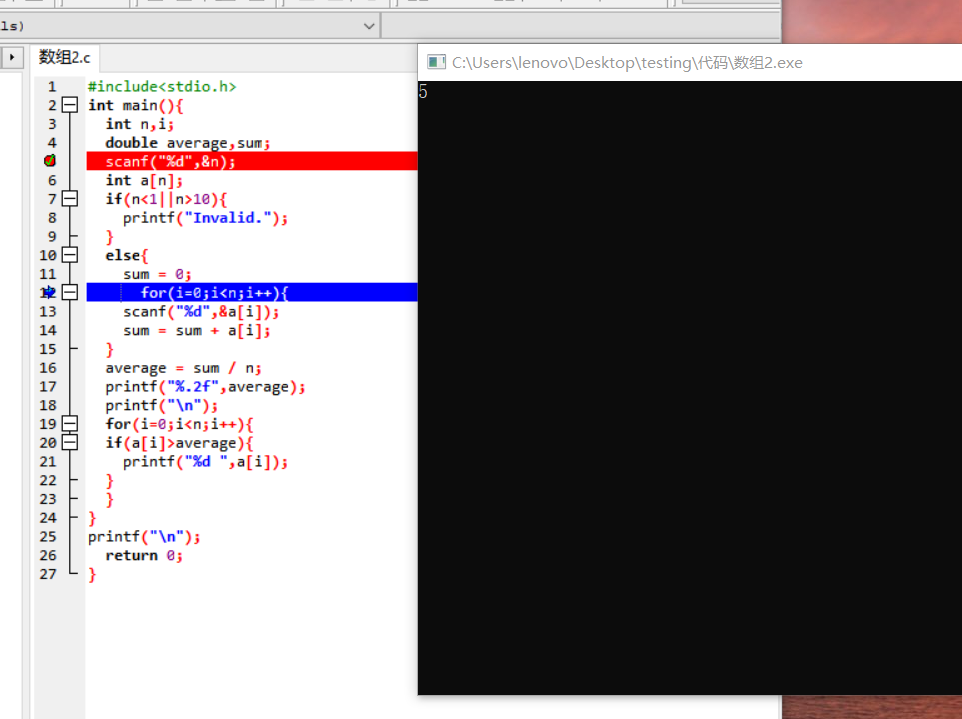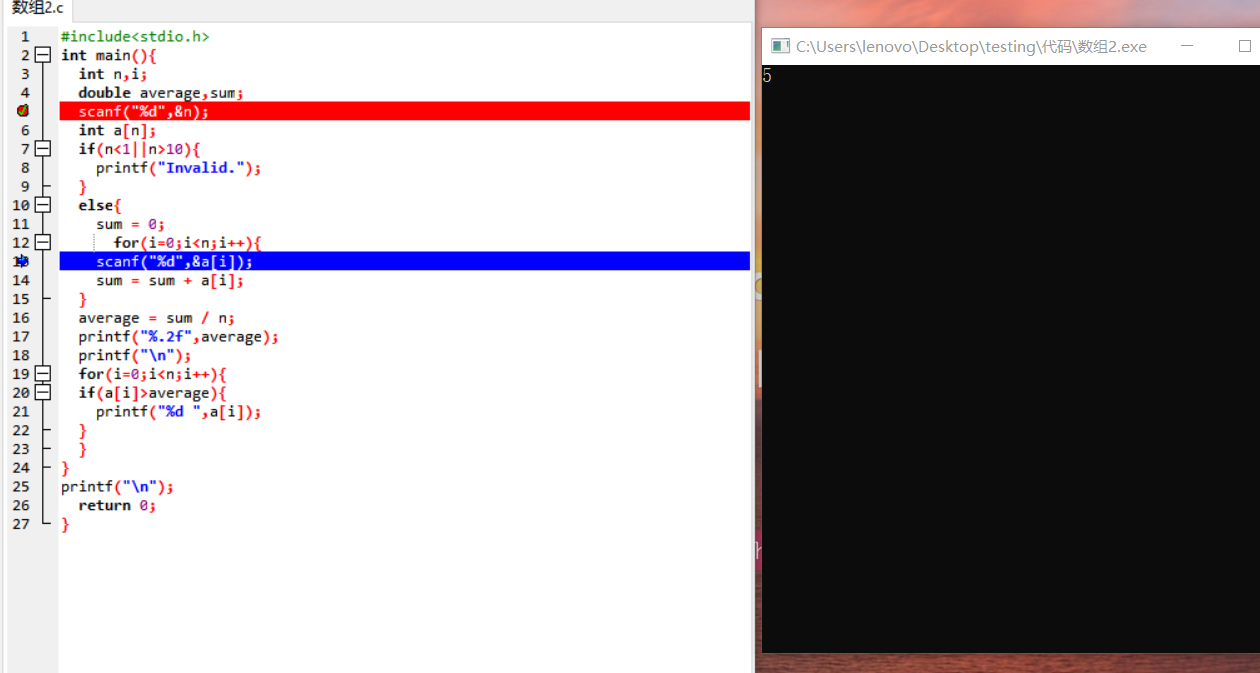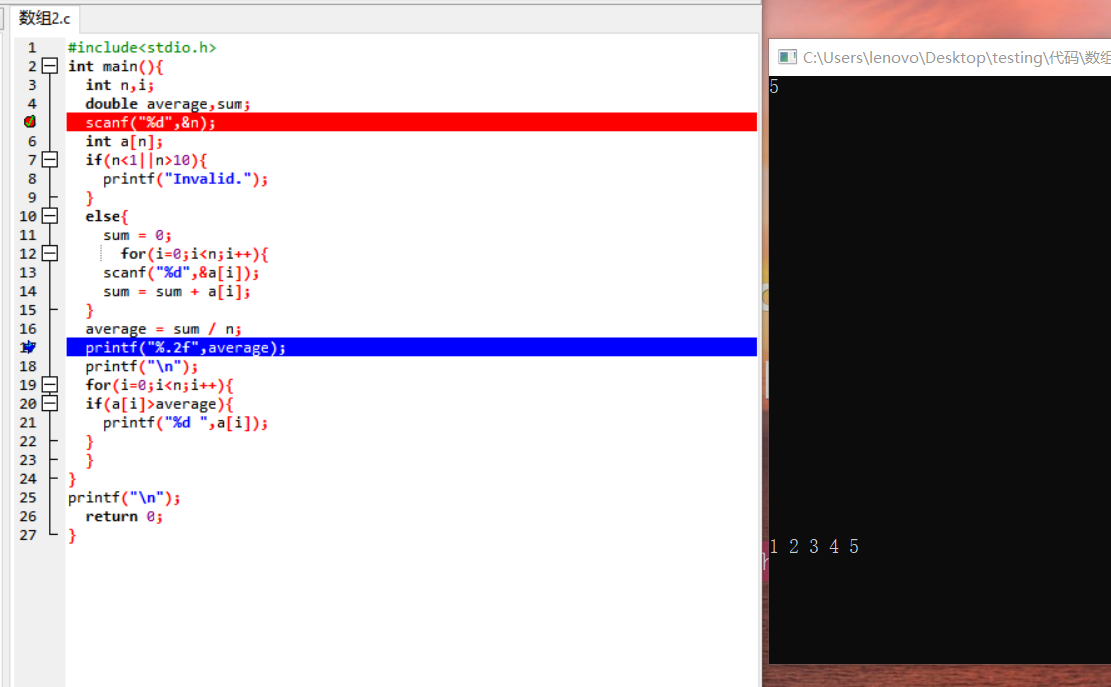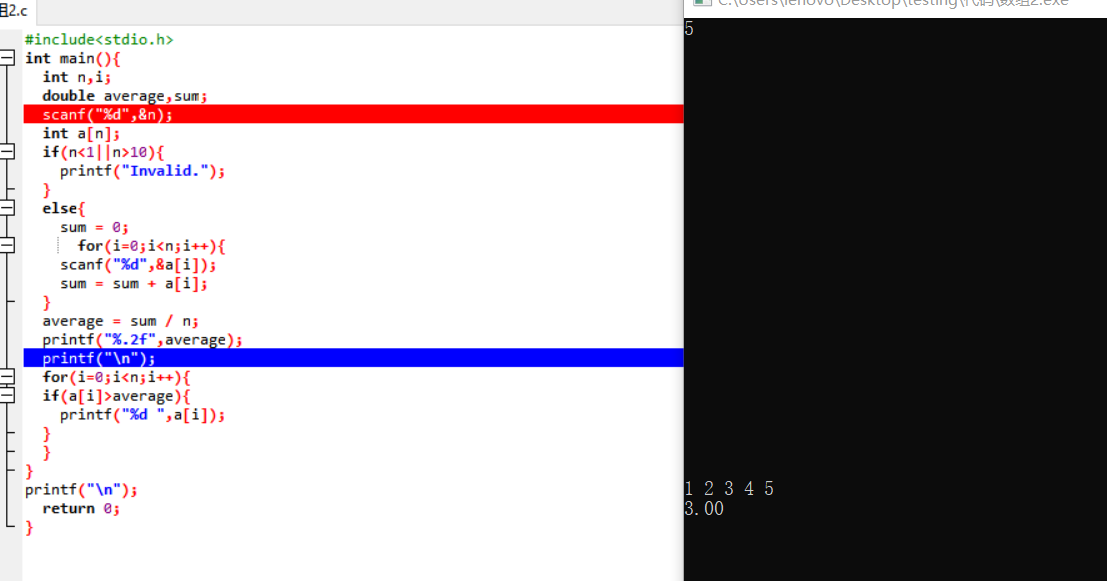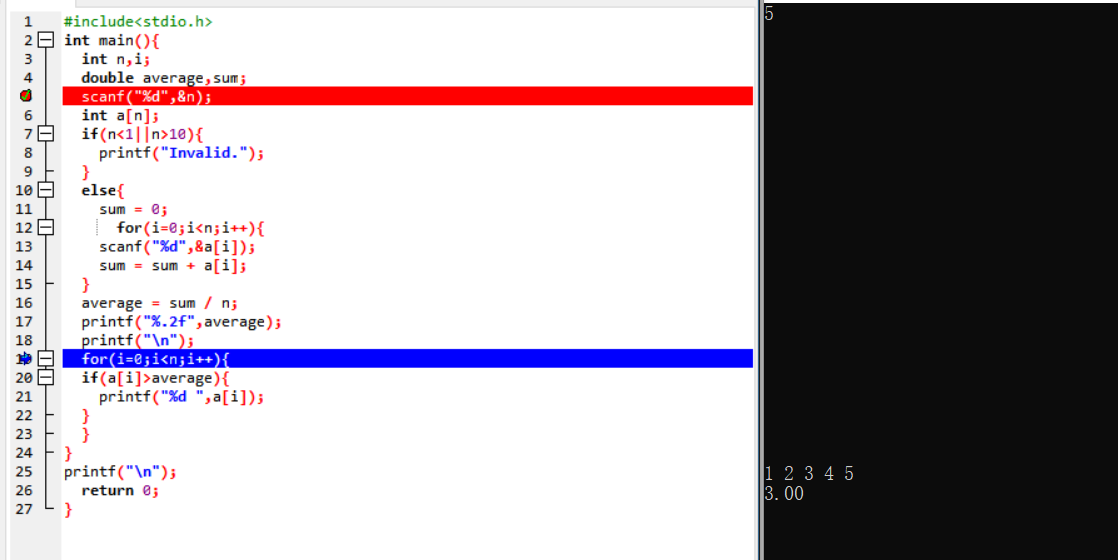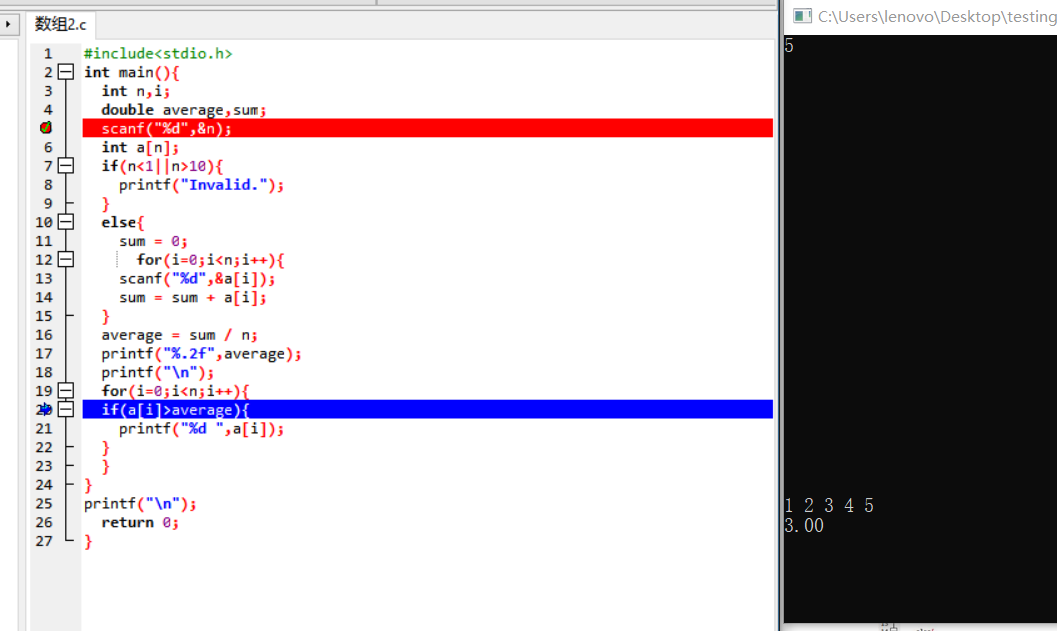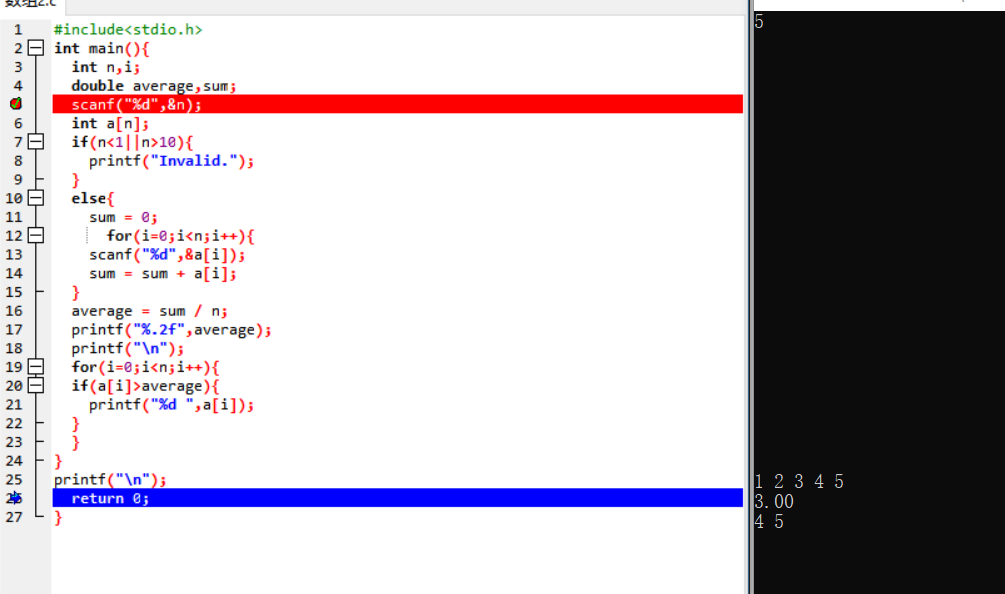1）7-2 求最大值及其下标 （20 分）

1）实验代码

#include <stdio.h>
int main(){
int n,i,j;
int a;
scanf("%d",&n);
for(i=0;i<n;i++){
scanf("%d",&a[i]);
}
for(j=0;j<n;j++){
if(a<a[j]){
a=a[j];
i=j;
}
if(a==a[j]){
a=a[j];
if(i>j){
i=j;
}
}
}
printf("%d %d",a[i],i);
return 0;
}

2）解题思路

3）流程图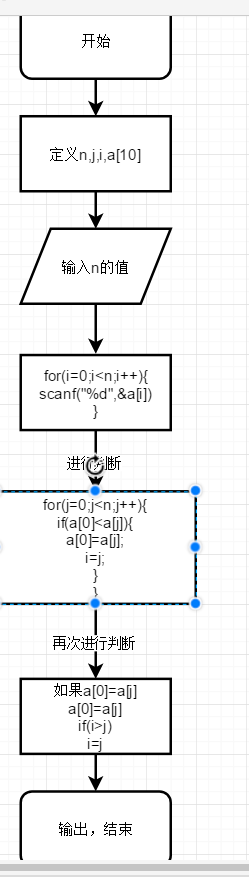4）调试过程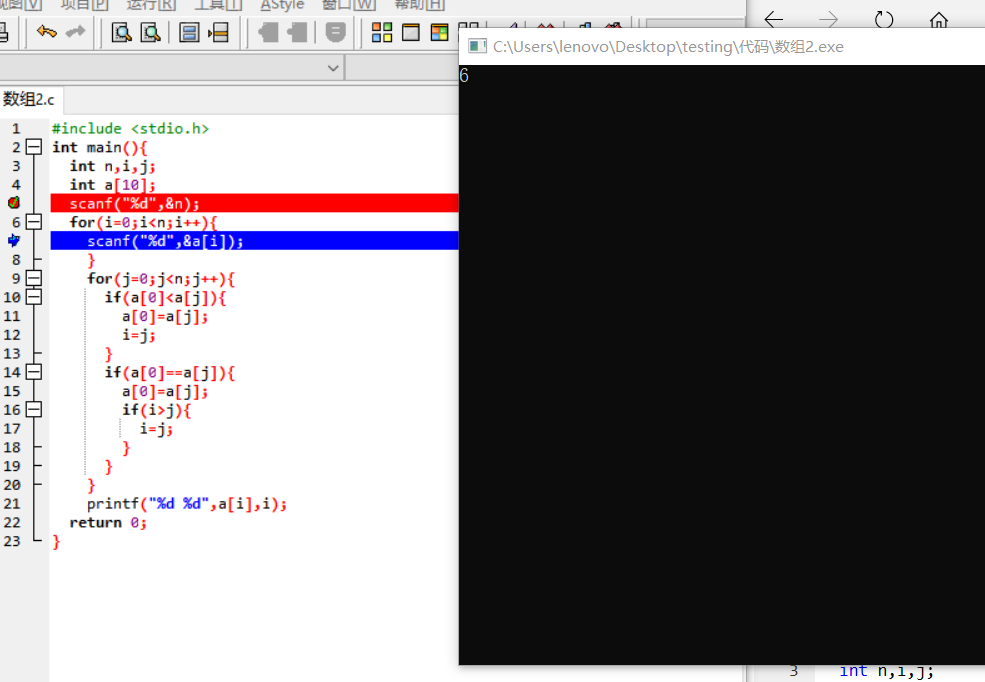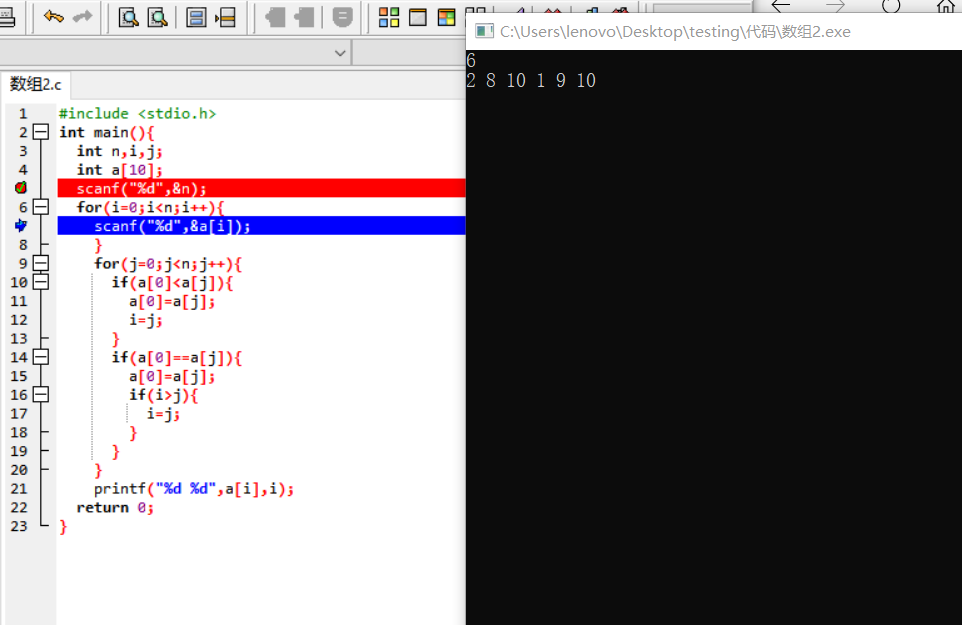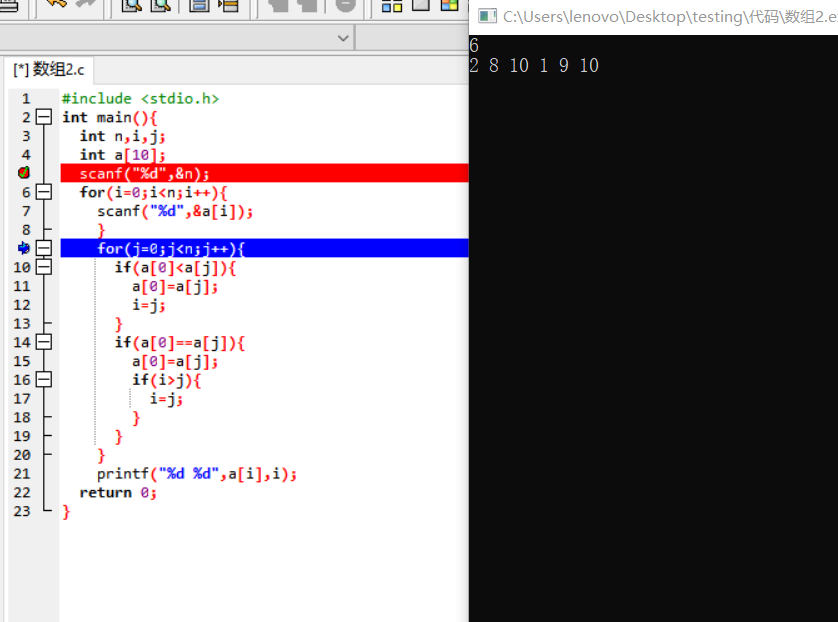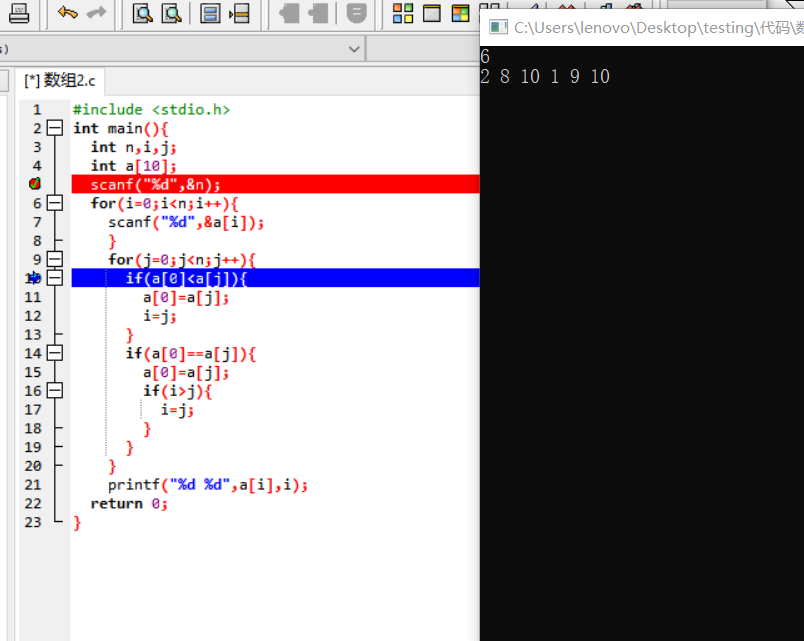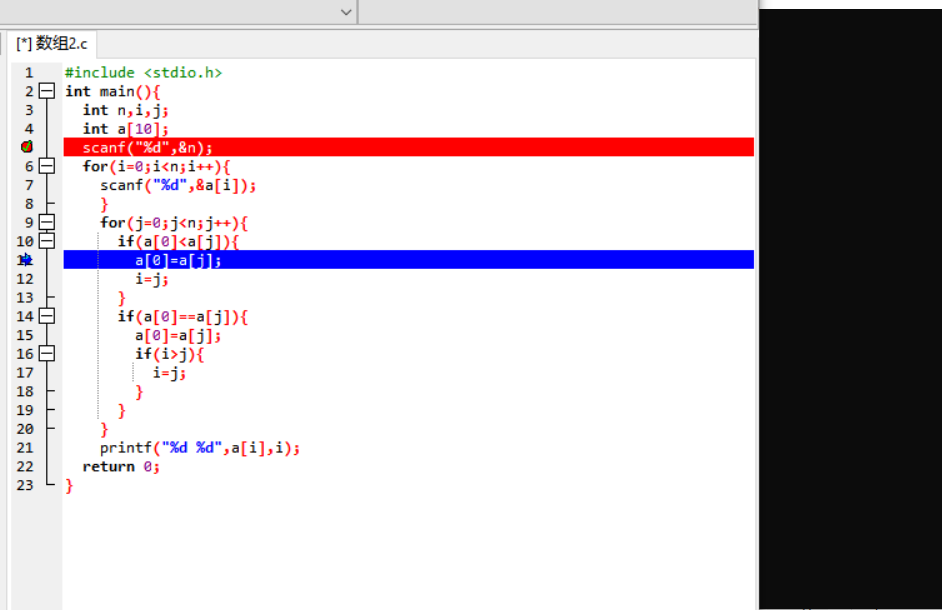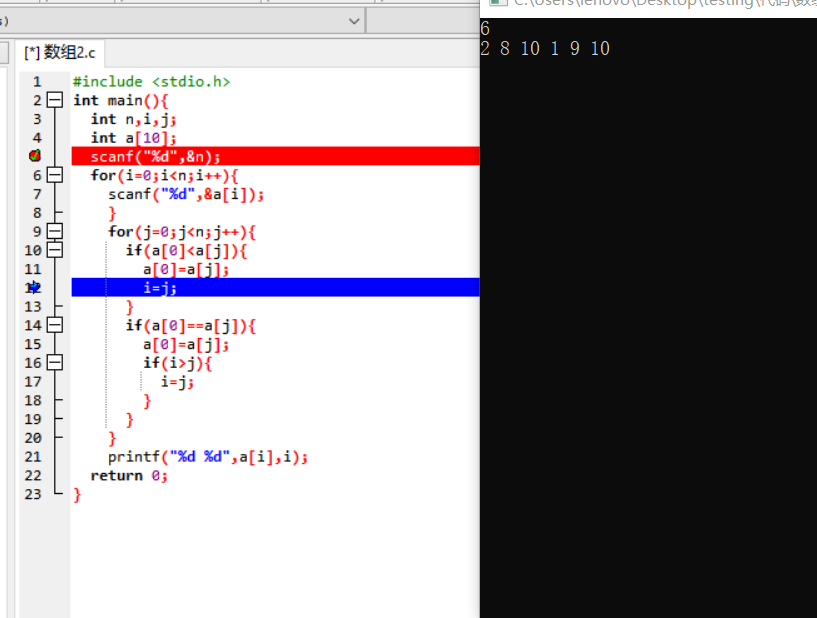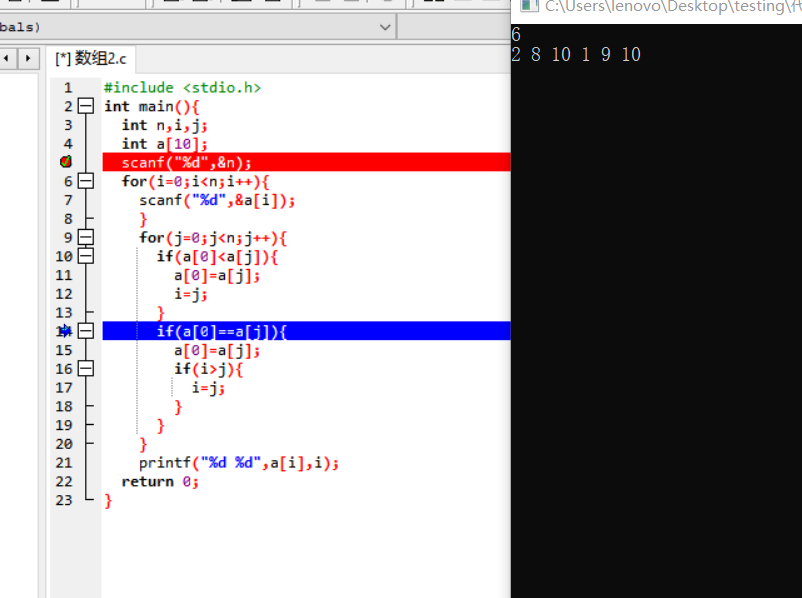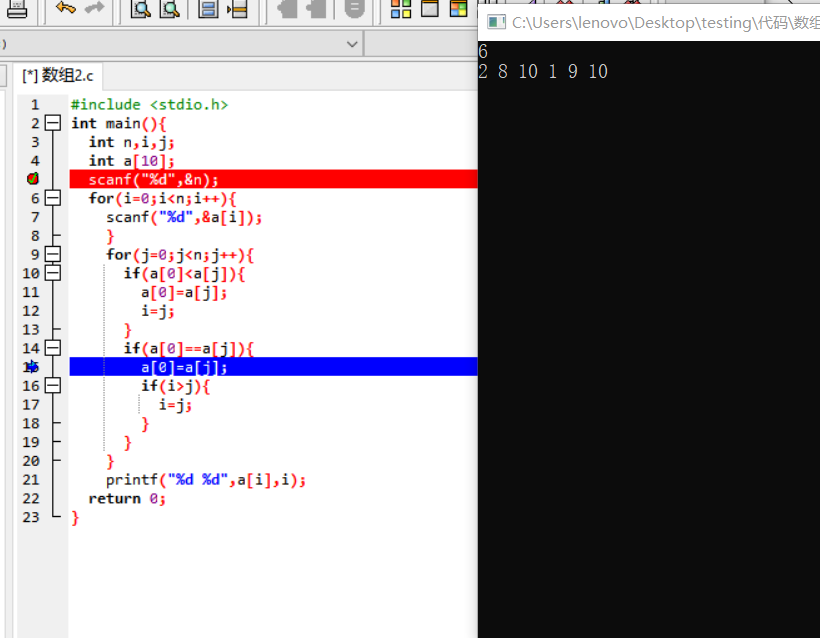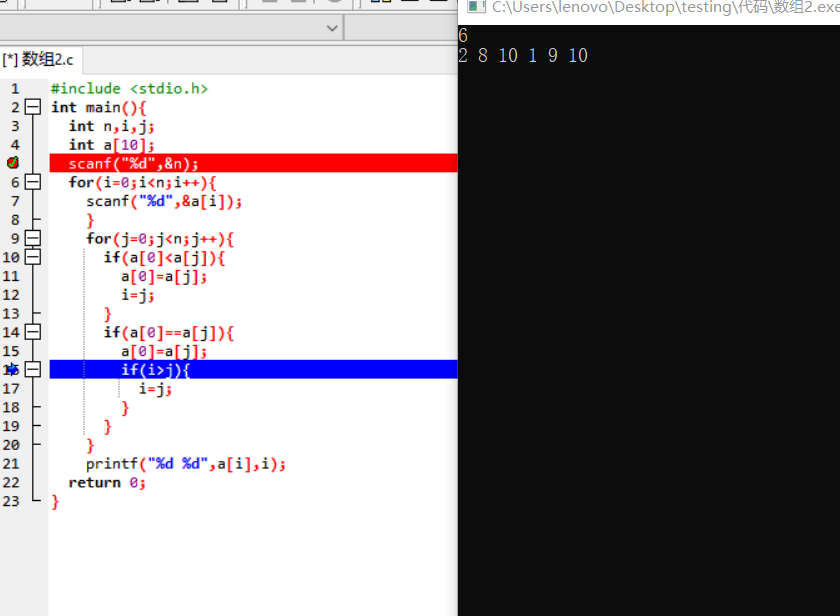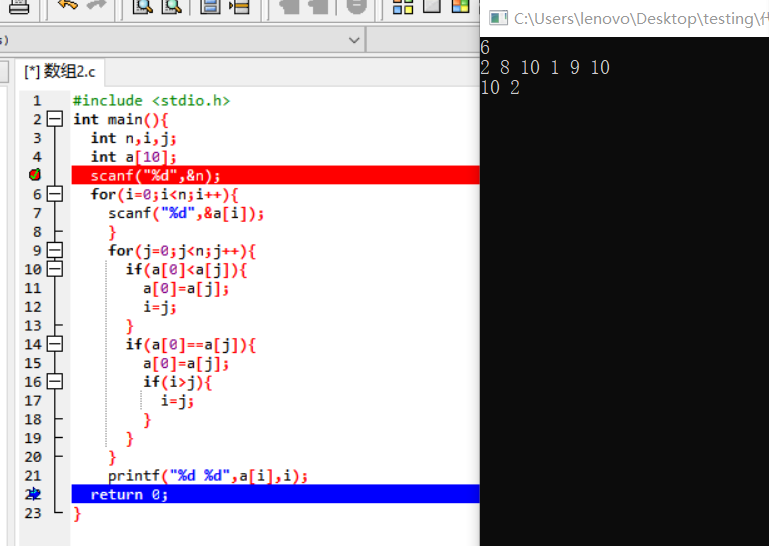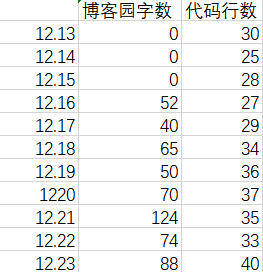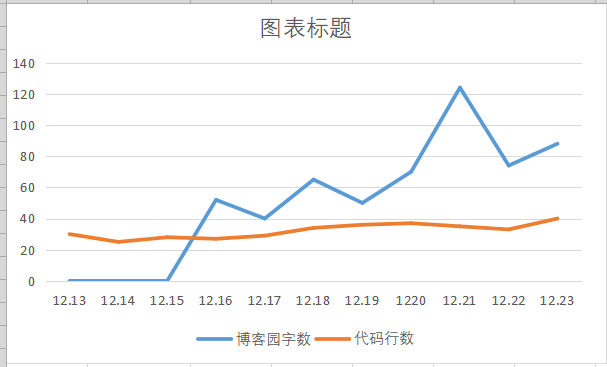posted on 2018-12-25 21:44  宋博文  阅读(216)  评论(8编辑  收藏  举报